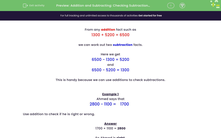# Use Addition to Check Subtractions

In this worksheet, students will use additions to check subtractions. Simple four-digit numbers are used.Key stage:  KS 2

Curriculum topic:   Number: Addition and Subtraction

Curriculum subtopic:   Estimate Calculations and Check Answers

Difficulty level:#### Worksheet Overview

From any addition fact such as

1,300 + 5,200 = 6,500

we can work out two subtraction facts.

Here we get:

6,500 - 1,300 = 5,200

and

6,500 - 5,200 = 1,300

This is handy because we can use additions to check subtractions.

Example 1

Ahmed says that

2,800 - 1,100 =
 1,700

Use addition to check if he is right or wrong.

1,700 + 1,100 = 2,800

So Ahmed is right.Example 2

Bill says that

6,500 - 3,700 =
 3,200

Use addition to check if he is right or wrong.

3,200 + 3,700 = 6,900 not 6,500

So Bill is wrong.Let's have a go at some questions on this now.

### What is EdPlace?

We're your National Curriculum aligned online education content provider helping each child succeed in English, maths and science from year 1 to GCSE. With an EdPlace account you’ll be able to track and measure progress, helping each child achieve their best. We build confidence and attainment by personalising each child’s learning at a level that suits them.

Get started